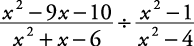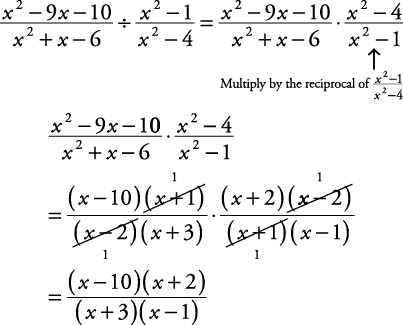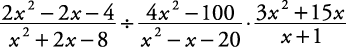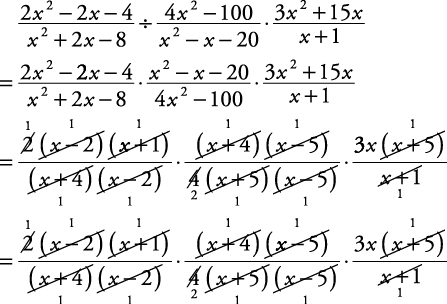## Dividing Rational Expressions

Just as in arithmetic, division of fractions involves multiplying by the reciprocal of the divisor.

##### Example 1

Simplify.If this last answer is multiplied out, it is##### Example 2

Simplify.

Since only the middle expression is doing the dividing, it is the only one whose reciprocal is used.Back to Top
A18ACD436D5A3997E3DA2573E3FD792A